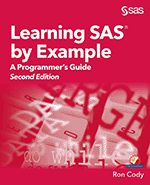I often get asked for programming tips. Here, I share three of my favorite tips for beginners.

### Tip #1: COUNTC and CATS Functions Together

The CATS function concatenates all of its arguments after it strips leading and trailing blanks. The COUNTC function counts characters. Together, they can let you operate on several separate variables as if they were a single string. To demonstrate, here is a program to count the number of Y's and N's in a five question survey.

```*Old way Make an array of the questions, initialize counters, count the Y's and N's in a DO loop;;   data survey; input (Ques1-Ques5)(\$1.); array Ques; Num_Yes = 0; Num_No = 0; do i = 1 to 5; if upcase(Ques[i]) = 'Y' then Num_Yes + 1; else if upcase(Ques[i]) = 'N' then Num_No + 1; end; drop i; datalines; yYnNY yyYYn NNnnn ;```
```*New way Use the CATS function to concatenate the 5 questions. Then, use the COUNTC function to count the number of Y's or N's in the string, using the ignore case modifier.;   data survey; input (Ques1-Ques5)(\$1.); Num_Yes = countc(cats(of Ques1-Ques5),'y','i'); Num_No = countc(cats(of Ques1-Ques5),'n','i'); datalines; yYnNY yyYYn NNnnn ;```

### Tip #2: Use CALL SORTN to Save Lots of Coding

The CALL SORTN routine sorts values WITHIN an observation. One way to use this call routine is:
CALL SORTN(of Var1-Var10);

After the call, the values of Var1 to Var10 will be in ascending order. Here is an example where you use this call routine to compute the mean of the highest 8 quiz scores out of a total of 10.

```*Tip 2; *Use CALL SORTN to compute the mean of the 8 highest scores (out of 10); data grade; input Score1-Score10; call sortn(of Score1-Score10); Mean_8 = mean(of Score3-Score10); /* alternative program call sortn(of Score10-Score1); *Descending sort; Mean_8 = mean(of Score1-Score8); */ datalines; 80 90 100 50 92 90 79 98 100 55 ;```

### Tip #3: Cody's Law of SAS Programming

If you find yourself writing a very tedious SAS program--STOP. Take a walk, talk to a colleague, then ask yourself, “Does SAS have some tool to make this program less tedious?” Perhaps an ARRAY, or a MACRO, or a FUNCTION can help. Sometimes brute force programming is necessary, but very often, there is a SAS tool that will make for an easier and more elegant program.

I hope you find these helpful! For more tips and example code please check out my new book, the new edition of Learning SAS® by Example: A Programmer’s Guide. All the programs and examples described are fully compatible with SAS University Edition too. Happy programming!

Share